# Objective 5. Use Financial Functions

Financial functions perform common business calculations such as calculating a loan payment on a vehicle or calculating how much to save each month to buy something. Financial functions commonly involve a period of time such as months or years.

Activity 3.11. Designing a Loan Worksheet

1.

Start Excel and close the task pane. From the File menu, display the Save As dialog box, navigate to the location where you are storing your projects for this chapter, and then in the File name box, type 3C_Loan_Payment_Firstname_Lastname Click Save or press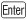.

2.

Widen column A to 160 pixels and column B to 100 pixels. In the range A2:B5, enter the following titles and data. Recall that you can format the numbers as you type by typing them with their symbols as shown:

 Amount of Loan \$180,000 Period (years) 3 Interest rate (per year) 7% Payment (per month)

3.

In cell A1, type Truck Loan Options Merge and center the title in the range A1:B1, change the font to Arial Black, and then change the font size to 14. Rename the worksheet tab Truck Loan and then Save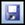your workbook. Compare your screen with Figure 3.28.

Figure 3.28.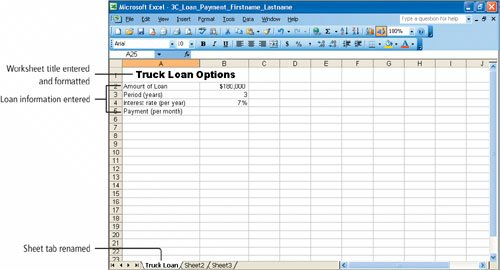Activity 3.12. Applying the Financial Function PMT

When you borrow money from a bank, the amount charged to you for your use of the borrowed money is called interest. Loans are typically made for a period of years, and the interest that must be paid is a percentage of the loan amount that is still owed. In Excel, this percentage is called the rate. The initial amount of the loan is called the Present value (Pv), and the number of time periodsnumber of paymentsis abbreviated nper. The value at the end of the time periods is the Future value (Fv), which is usually zero for loans.

In this activity, you will calculate the monthly payments the college will have to make to finance the purchase of eight new pickup trucks, the total cost of which is \$180,000. You will calculate the monthly payments, including interest, for a three-year loan at an annual interest rate of 7.0%. To stay within Darron's budget, the monthly payment must be under \$4,500.

 1. Click cell B5. On the Formula Bar, click the Insert Function button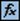. Click the Or select a category arrow, and then within the Financial category, scroll as necessary and click PMT. Click OK to display the Function Arguments dialog box for the PMT function. If necessary, drag the dialog box to the right side of your screen so that you can view columns A:B. The PMT function calculates the payment for a loan based on constant payments and at a constant interest rate. To complete the PMT function, you must first determine the total number of loan payment periods (months), which is 12 months x 3 years, or 36 months. 2. With your insertion point positioned in the Rate box, type b4/12 Alternatively, click cell B4 and then type /12. Excel will divide the annual interest rate of 7%, which is 0.070 in decimal notation, located in cell B4 by 12 (months), which will result in a monthly interest rate. When borrowing money, the interest rate and number of periods is quoted in years. The payments on a loan, however, are usually made monthly. Therefore, the number of periods, which is stated in years, and the annual interest rate must be changed to a monthly equivalent in order to calculate the monthly payment amount. You can see that calculations like these may be made as part of the argument in a function. 3. Press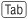to move the insertion point to the Nper box. In the lower portion of the dialog box, notice that Nper is the total number of payments for the loan (number of periods). Type b3*12 to have Excel convert the number of years in the loan (3) to the total number of months. Recall that the PMT function calculates a monthly payment. Thus, all values in the function must be expressed in months. 4. Pressto move to the Pv box and type b2 Pv represents the present valuethe amount of the loan before any payments are madein this instance \$180,000. 5. In cell B5 and on the Formula Bar, notice that the arguments that comprise the PMT function are separated by commas. Notice also, in the Function Arguments dialog box, that the value of each argument displays to the right of the argument box. Compare your screen with Figure 3.29.   Figure 3.29.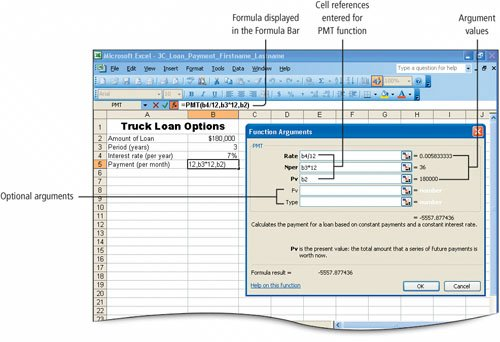NoteOptional Arguments The PMT function has two arguments not indicated by bold; these are optional. The Future value (Fv) argument assumes that the unpaid portion of the loan should be zero at the end of the last period. The Type argument assumes that the payment will be made at the end of each period. These default values are typical of most loans and may be left blank. 6. In the displayed dialog box, click OK. The monthly payment amount, (\$5,557.88), displays in cell B5. The amount displays in red and in parentheses to show that it is a negative number, a number that will be paid out. This monthly payment of \$5,557.88 is over the budget of \$4,500 per month that Darron has in mind. 7. Click in the Formula Bar, and then use the arrow keys on the keyboard as necessary to position the insertion point between the equal sign and PMT. Type - to insert a minus sign into the formula and press. Saveyour workbook.   The monthly payment amount, \$5,557.88, displays in cell B5 as a positive number, which is more familiar and less distracting to work with.

[Page 818 (continued)]

### Objective 6 Use Goal Seek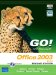GO! with Microsoft Office 2003 Brief (2nd Edition)
ISBN: 0131878646
EAN: 2147483647
Year: 2004
Pages: 448